Physical Chemistry : Acid-Base Equilibrium and Titration

Example Questions

Example Question #1 : Acid Base Equilibrium And Titration

A 50mL solution of 0.2M hydrochloric acid is titrated with a 0.2M sodium hydroxide solution. What is the pH of the solution after 48mL of the sodium hydroxide solution has been added?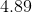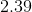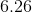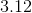Explanation:

Since a strong acid is being titrated with a strong base, we can simply subtract how many moles of base have been added from how many moles of acid were originally present.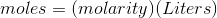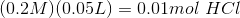Using the same equation, we found we have added 0.0096 moles of sodium hydroxide.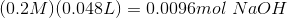Subtracting this amount from the hydrochloric acid leaves us with 0.0004 moles of acid.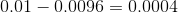Keep in mind that we must divide this molar amount by the new volume, after the base has been added.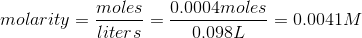Since this is a strong acid, we can simply take the negative log of this concentration, and are left with a pH of 2.39.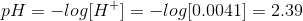Example Question #2 : Acid Base Equilibrium And Titration

A 50mL solution of 0.2M hydrochloric acid is titrated with a 0.2M sodium hydroxide solution. What is the pH when 50mL of sodium hydroxide has been added?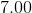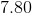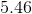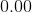Explanation:

When the molar amount of acid equals the molar amount of base, the solution has reached what is known as the equivalence point. When a strong acid is titrated with a strong base, such as in this example, the two agents will neutralize each other completely. Because the conjugate base for the acid is incredibly weak, it will not manipulate the pH. As a result, the pH at this equivalence point is 7.00.

Example Question #1 : Acid Base Equilibrium And Titration

A 50mL 0.2M hydrofluoric acid solution is titrated with a 0.2M sodium hydroxide solution. What is the pH of the solution when 20mL of base has been added?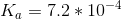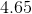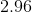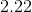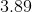Explanation:

Since hydrofluoric acid is a weak acid, we need to consider how much of the conjugate base is created when the base neutralizes the acid. We can start by determining how many moles of acid are present initially.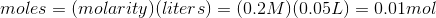Using the same equation, we find that only 0.004 moles of base have been added.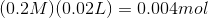After the acid and base cancel each other out, we are left with 0.006 moles of acid and 0.004 moles of the conjugate base. This is based on the neutralization equation: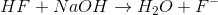(For simplicity, the bystander sodium ion has been omitted, although it is present).

Now that we know the amount of acid and conjugate base, we can solve for the pH using the Henderson-Hasselbalch equation.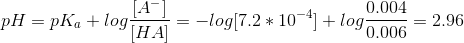Example Question #4 : Acid Base Equilibrium And Titration

A 50mL 0.2M hydrofluoric acid solution is titrated with a 0.2M sodium hydroxide solution. What is the pH of the solution when 50mL of sodium hydroxide has been added?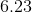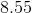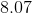Explanation:

This question is not as easy as just assuming that the pH is 7 because there are equal amounts of acid and base. The reason is because although the acid and base have neutralized each other, there is still the issue of the fluoride ion acting as a conjugate base. The equation for this reaction is:(The sodium ion has been omitted, as it is simply a spectator ion).

By converting each amount of acid and base to moles, we can find that 0.01 moles of each has been added, neutralizing each other in the process. According to the equation, that will leave us with 0.01 moles of fluoride ions. In order to find the concentration, we simply divide this by the new volume after all of the base has been added: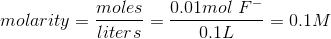Next, we can determine the base dissociation constant for the fluoride ion by using the equation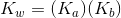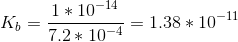Finally, we use an ICE table in order to determine the hydroxide concentration in the solution.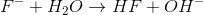The fluoride ion concentration will decrease by, and both the hydroxide and hydrofluoric acid concentrations will increase by. This leaves us with the equilibrium expression: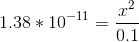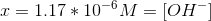Using the pH equation, we determine the new pH of the system to be 8.07.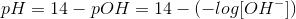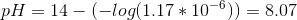Example Question #1 : Acid Base Equilibrium And Titration

A 50mL 0.2M hydrofluoric acid solution is titrated with a 0.2M sodium hydroxide solution. What is the pH of the solution when 60mL of base has been added?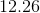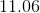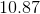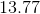Explanation:

Since there is now more strong base than weak acid in the solution, the remaining amount of strong base alone will dictate the pH.

By converting concentration to moles, we can determine that we started with 0.01 moles of weak acid.Using the same equation, we can determine that 0.012 moles of base was added.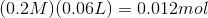After neutralization, that leaves us with 0.002 moles of strong base. This strong base is the only value we will use to determine the pH. Since the final volume is 110mL, the concentration of remaining sodium hydroxide is calculated as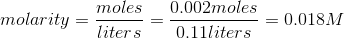Finally, we can solve for the pH by first finding the pOH, then subtracting from 14.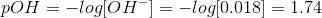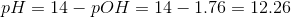Example Question #1 : Acid Base Equilibrium And Titration

A researcher prepares two solutions. In solution A, he adds equal volumes of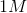hydrochloric acid and sodium hydroxide. In solution B, he adds equal volumes ofacetic acid and sodium hydroxide. What can you conclude about the relative concentrations of the salt produced in solution A and solution B?

Solution B will have a higher concentration of salt

Both will solutions will have equal concentration of salt

Solution A will have a higher concentration of salt

Cannot be determined without knowing the volume of the solutions

Solution A will have a higher concentration of salt

Explanation:

A generic acid-base reaction involves the production of salt and water. The amount of products depends on the strength of the reactants. Recall that a strong acid completely dissociates into hydrogen ions and conjugate base in water. Similarly, a strong base completely dissociates into conjugate acid and hydroxide ions in water. A salt (like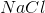) is formed from the conjugate base (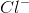from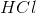) and conjugate acid (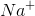from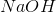); therefore, to get the highest yield of salt we need complete dissociation of acid and base into their respective conjugates. Since they completely dissociate and form conjugates, strong acid and strong base will yield the highest concentration of salt (in this question solution A).

Solution B will also form salt; however, since acetic acid is a weak acid only few molecules of conjugate base (acetate) will be produced, thereby decreasing the amount of salt produced.

Example Question #1 : Acid Base Equilibrium And Titration

Which of the following are true regarding acid-base equilibrium?

I. The pH of a weak acid/strong base solution is higher than a weak acid/weak base solution

II. The pKa of an acid determines how much hydrogen ion is produced in solution

III. A strong acid/strong base solution will have a hydroxide ion concentration of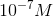I, II, and III

I

II and III

I and II

I, II, and III

Explanation:

The pH is a measure of the concentration of hydrogen ions in solution. An increase in pH corresponds to lower hydrogen ion concentration whereas a decrease in pH corresponds to higher hydrogen ion concentration. Recall that a strong base dissociates completely into conjugate acid and hydroxide ions whereas a weak acid doesn’t dissociate completely into conjugate base and hydrogen ions. Since there is an excess of hydroxide ions in a weak acid/strong base solution, the hydroxide ions will react with and consume the hydrogen ions from weak acid. This will decrease the hydrogen ion concentration and, subsequently, increase the pH. On the other hand, a weak base doesn’t produce as many hydroxide ions; therefore, not as much hydrogen ions will be consumed (pH will be decreased).

The pKa is a measure of the strength of an acid. The lower the pKa the stronger the acid. The extent of dissociation of acid into hydrogen ions and conjugate base depends on the strength; therefore, pKa determines how much hydrogen ion will be produced.

A strong acid and strong base will completely dissociate into their respective conjugates. This means that if equal amounts of strong acid and strong base are added, equal amounts of hydrogen ions and hydroxide ions are produced. Since there are equal amounts of them, hydrogen ions and hydroxide ions will react with each other and form water. Recall that water has a pH of 7; therefore, a strong acid/strong base solution will always have a pH of 7 (if equal amounts of strong acid and strong base are added). The definition of pH is: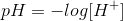If we solve for hydrogen ion concentration: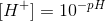Therefore, the hydrogen ion concentration in a strong acid/strong base solution is: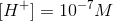The relationship between hydrogen ion concentration and hydroxide ion concentration is: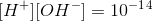If we solve for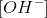: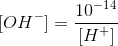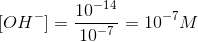Example Question #1 : Acid Base Equilibrium And Titration

During titration, you observe that the buffer region occurs at around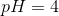. Which of the following might be true regarding this solution?

The solution contains acetic acid and ammonia

The solution is made up of hydrochloric acid and acetic acid

The solution contains a strong base

The solution contains hydrochloric acid and ammonia

The solution contains a strong base

Explanation:

The buffer region occurs when the pH of the solution equals the pKa of the acid. This means that the pKa of the acid is 4; therefore, this acid is a weak acid. Recall that buffer regions occur during titration when a weak component (acid or base) is added to a strong component (acid or base). For example, buffer regions can be seen when a weak acid is added to a strong base. Since we already determined that the acid is weak, the other component in the solution must be a strong base. Note that titration with two strong agents (strong acid and strong base) does not produce a buffer region.

Example Question #1 : Acid Base Equilibrium And Titration

Compared to a weak acid-strong base titration, a strong acid-strong base titration has a __________ pH at the equivalence point and a __________ pH at the endpoint.

lower . . . higher

lower . . . lower

higher . . . lower

higher . . . higher